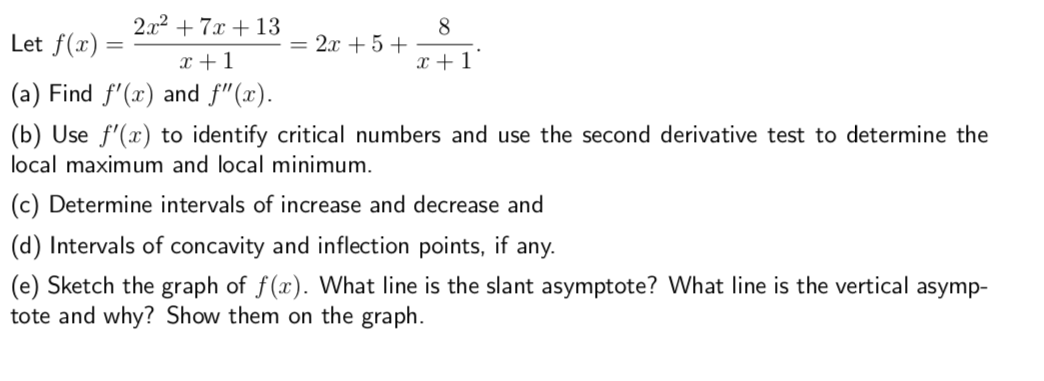# Let2x2 +7x +13r+112+5+1(a) Find f(x) and f"(x).(b) Use f(x) to identify critical numbers and use the second derivative test to determine thelocal maximum and local minimum(c) Determine intervals of increase and decrease and(d) Intervals of concavity and inflection points, if any.(e) Sketch the graph of f(x). What line is the slant asymptote? What line is the vertical asymp-tote and why? Show them on the graph

Question
11 viewshelp_outlineImage TranscriptioncloseLet 2x2 +7x +13 r+1 12+5 +1 (a) Find f(x) and f"(x). (b) Use f(x) to identify critical numbers and use the second derivative test to determine the local maximum and local minimum (c) Determine intervals of increase and decrease and (d) Intervals of concavity and inflection points, if any. (e) Sketch the graph of f(x). What line is the slant asymptote? What line is the vertical asymp- tote and why? Show them on the graph fullscreen
check_circle

Step 1

We use the power rule of differentiation which is derivative if x^n is nx^(n-1)  to find f'(x) and f''(x).

Step 2

2) We now that the critical numbers are the solutions of f'(x)=0, so we set f'(x)=0 and find the value of satisfyingf'(x)=0

Therefore, critical numbers are 1 and -3.

Step 3

Since, at x=1, f''(x)>0, and at x=-3, f''(x)<0

Therrefore, ...

### Want to see the full answer?

See Solution

#### Want to see this answer and more?

Solutions are written by subject experts who are available 24/7. Questions are typically answered within 1 hour.*

See Solution
*Response times may vary by subject and question.
Tagged in

### Derivative